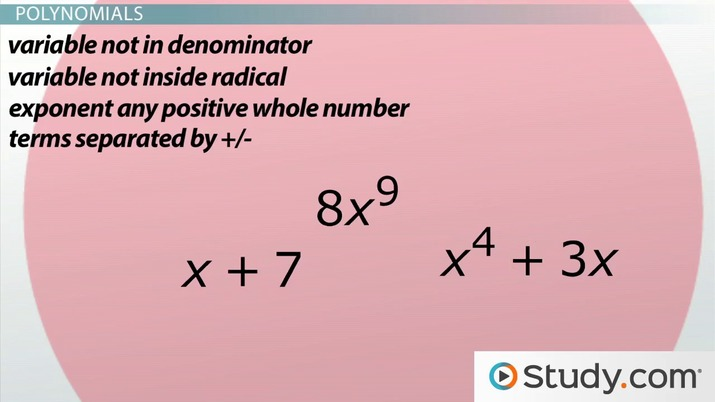# What are Polynomials, Binomials, and Quadratics?

Lesson Transcript
Instructor: Yuanxin (Amy) Yang Alcocer

Amy has a master's degree in secondary education and has been teaching math for over 9 years. Amy has worked with students at all levels from those with special needs to those that are gifted.

Polynomials, binomials, and quadratics refer to the number of terms an expression has in math. Study the definition and the three restrictions of polynomials, as well as the definitions of binomials and quadratics. Updated: 09/27/2021

This is one area of math where you can relax a little bit. Polynomials, binomials, and quadratics are not tricky things you have to learn. They are names of mathematical expressions that are easy to work with. Knowing what they have in common and how you can identify each of these will help you work through your math problems easier. In this video, we're going to go through what each of them is and how you can identify each.

The first thing I can tell you about all three of these is that they are all polynomials. So, picture a big balloon with all your polynomials floating around inside. Inside the balloon, you will find two smaller balloons with binomials and quadratics inside. Let's talk about polynomials and what makes them unique.An error occurred trying to load this video.

Try refreshing the page, or contact customer support.

Coming up next: How to Add, Subtract and Multiply Polynomials

### You're on a roll. Keep up the good work!

Replay
Your next lesson will play in 10 seconds
• 0:06 Polynomials,…
• 0:54 Polynomials
• 2:27 Binomials
• 3:59 Lesson Summary
Save Save

Want to watch this again later?

Timeline
Autoplay
Autoplay
Speed Speed

## Polynomials

Polynomials literally means many terms. They are easy to work with because they have three restrictions. What are the restrictions?

• First, the variable in each term cannot be a negative exponent.
• Second, the variable in each term cannot be in the denominator.
• Third, the variable in each term cannot be inside a radical. In other words, the variable's exponent must be a whole number.

If you see any of these, then you can zap them out of your balloon as they are not polynomials. Even though polynomials literally means many terms, you can have just one term or just two terms. A term means the multiplication of a number with a variable. The variable's exponent can be any positive whole number. All terms are separated by either a plus or a minus symbol. Of course, you can have as many terms as you want in a polynomial. There is no limit. All of these are polynomials that belong inside the balloon.

Notice how none of the variables have negative exponents, are in the denominator, or are inside a radical. All of the exponents for the variables are positive whole numbers. Also notice how all the terms are separated by either a plus or a minus. All of these polynomials are written in what is called standard form, starting with the largest exponent and working my way down. Now let's talk about binomials.

To unlock this lesson you must be a Study.com Member.

### Register to view this lesson

Are you a student or a teacher?